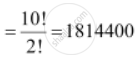CBSE (Arts) Class 11CBSE
Share

# In How Many Ways Can the Letters of the Word Permutations Be Arranged If The Words Start with P and End with S - CBSE (Arts) Class 11 - Mathematics

ConceptConcept of Permutations

#### Question

In how many ways can the letters of the word PERMUTATIONS be arranged if the words start with P and end with S

#### Solution

In the word PERMUTATIONS, there are 2 Ts and all the other letters appear only once

If P and S are fixed at the extreme ends (P at the left end and S at the right end), then 10 letters are left.

Hence, in this case, required number of arrangementsIs there an error in this question or solution?

#### APPEARS IN

NCERT Solution for Mathematics Textbook for Class 11 (2018 to Current)
Chapter 7: Permutations and Combinations
Q: 11.1 | Page no. 148

#### Video TutorialsVIEW ALL 

Solution In How Many Ways Can the Letters of the Word Permutations Be Arranged If The Words Start with P and End with S Concept: Concept of Permutations.
S